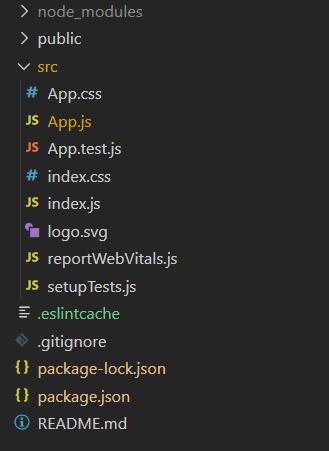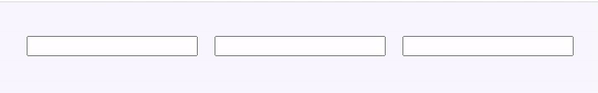# How to focus on the next field input in ReactJS ?

• Last Updated : 08 Apr, 2021

If we want to focus on the text input field when the current input field reaches its max character capacity, we have to find the next input HTML element and make it focus. Each time the user types in the current text field, we have to check whether the field has a max character that is specified. If yes, then we have to focus on the text input field. We can use focus() function to focus the particular input field.

Creating React Application:

Step 1: Create a React application using the following command:

`npx create-react-app foldername`

Step 2: After creating your project folder, i.e., foldername, move to it using the following command:

`cd foldername`

Project Structure: It will look like the following.Example: We can see in the code, whenever we type in the input field, the handleChange function will be called, and it will decide whether the next field should be the focus or not based on the number of characters typed in the current input field. If it reaches max character then it will find the next field and make it focus. This is how the next input field focuses when the current input field hits the max characters limit.

## App.js

 `import React from ``"react"``;`` ` `class App extends React.Component {``  ``render() {``    ``return` `(``      ``
``        ````      ``
``    ``);``  ``}``}`` ` `class InputFields extends React.Component {``  ``handleChange = (e) => {``    ``const { maxLength, value, name } = e.target;``    ``const [fieldName, fieldIndex] = name.split(``"-"``);`` ` `    ``let fieldIntIndex = parseInt(fieldIndex, 10);`` ` `    ``// Check if no of char in field == maxlength``    ``if` `(value.length >= maxLength) {`` ` `      ``// It should not be last input field``      ``if` `(fieldIntIndex < 3) {`` ` `        ``// Get the next input field using it's name``        ``const nextfield = document.querySelector(``          ```input[name=field-\${fieldIntIndex + 1}]```        ``);`` ` `        ``// If found, focus the next field``        ``if` `(nextfield !== ``null``) {``          ``nextfield.focus();``        ``}``      ``}``    ``}``  ``};`` ` `  ``render() {``    ``return` `(``      ``
``        ````        ````        ````      ``
``    ``);``  ``}``}``class InputFild extends React.Component {``  ``render() {``    ``return` `(``      ````    ``);``  ``}``}`` ` `export ``default` `App;`

Output:My Personal Notes arrow_drop_up